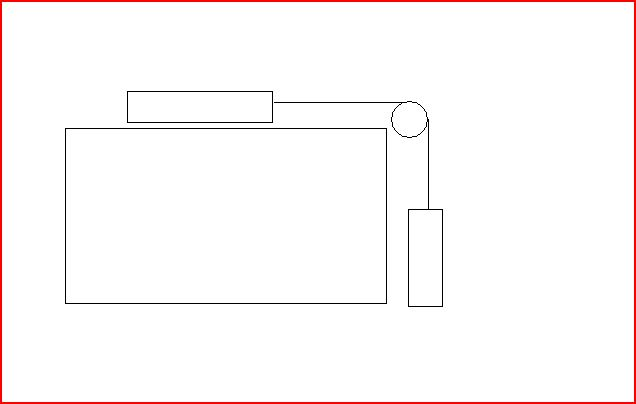# Power, torque, and velocity

## Homework Statement

A textbook of mass 1.99 kg rests on a frictionless, horizontal surface. A cord attached to the book passes over a pulley whose diameter is 0.150 m , to a hanging book with mass 2.97 kg . The system is released from rest, and the books are observed to move a distance 1.20 m over a time interval of 0.790 s .

A.What is the tension in the part of the cord attached to the textbook?
B.What is the tension in the part of the cord attached to the book?
C.What is the moment of inertia of the pulley about its rotation axis?

2. Homework Equations

F=ma
Torque= Iα
a=Rα
I=mR2

## The Attempt at a SolutionForces on book 1 (on top/textbook)

mg and Normal force cancel each other so the only force is to the right, Tension

t1=m1a

Forces on book 2 (hanging one)

-t2 + m2g= -m2a

Forces on wheel pulley

The string will create a torque in the clockwise direction

T = Iα
a = Rα
a/R = α

T = I (a/R)

I am stumped as what to do, I can find the t1 by using the second equation and solving for a in terms of t2 and plugging it back into the first one but then I would have another unknown variable.